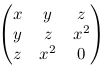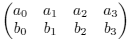Algebraic Geometry (NMAG401) - information about the course in winter semester 2017/2018.

## Basic information

The contents of the course and other basic information are available in the Student Information System.

The schedule (to be found also in the Student Information System):

• lectures on Fridays, 12.20pm-1.50pm in room K9,
• problem sessions on Fridays, 2.00pm-3.30pm in room K9.

## Credit

The credit will be granted for solved exercise problems. There will be three sets of the problems and they will appear here. Requested are at least 50 % of successfully solved problems handed in withing the deadlines.

### Problem set #1 (deadline for solutions: November 24)

1. A complex algebraic subset X ⊆ A2 is called a conic if it is of the form X = V(f) where f ∈ C[x,y] is a non-zero polynomial of total degree 2. Show that every irreducible conic is isomorphic either to V(y-x2) or to V(xy-1).
2. Let C be the field of complex numbers and consider the ring R = C[x,y,z]/(xz,yz) and the element f := y-z ∈ R. Show that the localized ring Rf (the element f is made invertible) is isomorphic to the ring C[x,y±1] × C[z±1].
3. Consider the subalgebra R ⊆ C[x,y] which consists of the polynomials only with terms of even total degree. Find an algebraic set X such that R is isomorphic to the coordinate ring C[X].

### Problem set #2 (deadline for solutions: December 22)

1. Show that the image of the polynomial map f: A1 → A3 given by f(t) = (t3, t4, t5) over the field of complex numbers is a subvariety of A3. Show further that it is precisely to set of zeros of the polynomial y2 - xz and the determinant of the matrix(hint: if x is non-zero, we can put t=y/x and divide the rows of the matrix by x).

Show finally that the polynomial p = x3 - yz vanishes on the image of f and find an expression of some power pe as an element of the ideal of C[x,y,z] generated by y2 - xz and the determinant (such an expression must exist by Hilbert's Nullstellensatz).

2. Consider the cubic plane curve X = V({x3 + y3 - 3x2 - 3y2 +3xy +1}) over the field of complex numbers. Find all singular points of X and find a concrete birational equivalence f: A1 → X (hint: stereographic projection from a singular point of X).
3. Let K be an algebraically closed field and I ⊆ K[x1, ..., xn] be an ideal. Show that the radical of I is equal to the intersection of the maximal ideals of K[x1, ..., xn] which contain I.

### Problem set #3 (deadline for solutions: January 12)

1. Let K be an algebraically closed field. We will define a map i from the set of 2-dimesional subspaces V ⊆ K4 to the projective space P5 as follows. We arbitrarily choose two linearly independent vectors v = (a0, a1, a2, a3) and w = (b0, b1, b2, b3) in V, we write the components into a 2x4 matrixand we compute (in any fixed order) the 6 determinants d0, d1, ..., d5 of all 2x2 submatrices. Then we define i to map V to (d0 : d1 : ... : d5).

1. Show that i is a well defined injective map.
2. Show that the image G of i is a projective variety given in P5 by a single quadratic equation.
2. Consider the map f: P1 → P3 given by f((s:t)) = (s3:s2t:st2:t3) over the field of complex numbers.
1. Show that f is a well-defined homomorphism of projective algebraic sets.
2. Find explicitly homogeneous polynomials f1, f2, ..., fr ∈ C[x0, x1, x2, x3] such that the image X of the map f satisfies X = Vproj(f1, f2, ..., fr).
3. Show that f: P1 → X is an isomorphism of projective algebraic sets.
3. Consider the 6-dimensional subspace V of C[x,y] consisting of all polynomials of total degree at most 2. Show that the irreducible conics in the complex affine space A2 are parametrized by a Zariski open subset of P5 (i.e. that the irreducible conics themselves form a quasi-projective variety).

## What has been lectured

A brief overview of what has been taught in individual lectures, including references to the literature, can be found below.

October 6, 2017
Affine algebraic sets, Zariski topology, the decomposition of a noetherian topological space to irreducible components, algebraic subsets of the affine plane (lecture notes, sec. 1; [Ful], sec. 1.2, 1.4 - 1.6, 6.1; [Ga], sec. 1.1, 1.3).
October 13, 2017
The decomposition of a noetherian topological space to irreducible components (proof), the ideal of a subset of an affine space and a characterization of irreducibility via prime ideals, polynomial maps and coordinate rings (lecture notes, sec. 1 and 2; [Ful], sec. 1.3, 1.5, 2.1 - 2.2; [Ga], sec. 1.3, 2.1).
October 20, 2017
The connection between polynomial maps and homomorphisms of coordinate rings, rational functions on varieties and function fields, rational maps between varieties (lecture notes, sec. 2; [Ful], sec. 2.2, 2.4, 6.6; [Ga], sec. 2.1, 2.3; [Sh], ch. 3).
October 27, 2017
The connection between rational maps with dense image and homomorphisms of function fields, birational equivalence, rational varieties (lecture notes, sec. 2; [Ful], sec. 6.6; [Ga], sec. 2.1, 2.3, 4.3; [Sh], ch. 3).
November 3, 2017
Rational plane curves, localization of rings (lecture notes, sec. 2 and 3; [Sh], ch. 3; [AM], ch. 3).
November 10, 2017
A geometric interpretation of the localization K[X] → K[X]f, radical ideals, Hilbert's Nullstellensatz (lecture notes, sec. 3; [Ful], sec. 1.7 - 1.10, 6.3; [Ga], sec. 1.2, 2.1; [AM], ch. 7).
November 24, 2017
Consequences of Hilbert's Nullstellensatz, an open basis for the Zariski topology, rational functions without poles over an algebraically closed field are polynomial ([Ful], sec. 1.7, 1.10, 2.4, 6.1; [Ga], sec. 1.2, 2.1).
December 1, 2017
Local rings at points of a variety and their properties, plane curves, singular and non-singular points, tangents ([Ful], sec. 2.4, 3.1; [Ga], sec. 4.4).
December 8, 2017
Discrete valuation rings and a characterisation of non-singular points on plane curves ([Ful], sec. 2.5, 3.2; [Ga], sec. 4.4).
December 15, 2017
Projective algebraic sets, projective Zariski topology, the correspondence with homogeneous ideals, a characterisation of irreducibility of projective algebraic sets ([Ful], sec. 2.6, 4.1, 4.2, 4.3; [Ga], sec. 3.1, 3.2).
December 22, 2017
Projective Nullstellensatz, homogeneous coordinate rings, function fields and regular functions on projective varieties, homomorphisms between projective varieties ([Ful], sec. 4.2, 6.3; [Ga], sec. 3.1 - 3.3).
January 5, 2018
Cartesian products of affine and projective varieties, the Segre embedding, quasi-projective varieties and homomorphisms between them, projective elimination theory, an exercise on blow-ups and resolution of singularities ([Ful], sec. 4.4, 6.1 - 6.3, 7.2; [Ga], sec. 2.3, 3.3, 3.4, 4.3).
January 12, 2018
Homomorphisms from projective varieties have a closed image, Krull dimension, the dimension of projective and affine spaces, the relation of the Krull dimension and the transcendence degree of the function field of a variety ([Ful], sec. 6.4, 6.5; [Ga], sec. 3.4, 4.1 - 4.2; [Sh], sec. 5.2 - 5.4, 6.1).

## Literature

I will write and update a draft version of lecture notes during the semester: [Full text in PDF]

As far as other sources are concerned, algebraic geometry has gradually grown to a very broad field with thousands of pages written about it from several points of view. To get an impression (or to get an idea where to go next for those interested in the topic), check this blog on mathoverflow.net. The core of the lecture is presented according to the following sources available in PDF:

 [Ga] A. Gathmann, Algebraic geometry, notes from a course in Kaiserslautern, 2002/2003. [Full text in PDF] [Ful] W. Fulton, Algebraic Curves (An Introduction to Algebraic Geometry), 2008. [Full text in PDF]

The lectures may also involve facts from the following (off-line) sources:

 [Sh] I. R. Shafarevich, Basic algebraic geometry 1, Varieties in projective space, 2. vyd., Springer-Verlag, Berlin, 1994. [AM] M. F. Atiyah, I. G. MacDonald, Introduction to Commutative Algebra, Addison-Wesley Publishing Co., 1969. [CLO] D. Cox, J. Little, D. O'Shea, Ideals, varieties, and algorithms, Second Edition, Springer, New York, 2005. [Na] M. Nagata, Local Rings, John Wiley & Sons, 1962. [Nee] A. Neeman, Algebraic and Analytic Geometry, LMS Lecture Note Series 345, Cambridge, 2007.